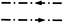International
Tables for
Crystallography
Volume A
Space-group symmetry
Edited by Th. Hahn

International Tables for Crystallography (2006). Vol. A. ch. 1.4, p. 7

## Section 1.4.1. Symmetry planes normal to the plane of projection (three dimensions) and symmetry lines in the plane of the figure (two dimensions)

Th. Hahna*

aInstitut für Kristallographie, Rheinisch-Westfälische Technische Hochschule, Aachen, Germany
Correspondence e-mail: hahn@xtal.rwth-aachen.de

### 1.4.1. Symmetry planes normal to the plane of projection (three dimensions) and symmetry lines in the plane of the figure (two dimensions)

| top | pdf |

Symmetry plane or symmetry lineGraphical symbolGlide vector in units of lattice translation vectors parallel and normal to the projection planePrinted symbolNone mAxial' glide planelattice vector normal to projection plane a, b or c
Double' glide plane (in centred cells only)e
Diagonal' glide planen
Diamond' glide plane (pair of planes; in centred cells only)along line parallel to projection plane, combined withnormal to projection plane (arrow indicates direction parallel to the projection plane for which the normal component is positive) d
For further explanations of the `double' glide plane e see Note (iv)below and Note (x)in Section 1.3.2.
Glide planes d occur only in orthorhombic F space groups, in tetragonal I space groups, and in cubic I and F space groups. They always occur in pairs with alternating glide vectors, for instanceand. The second power of a glide reflection d is a centring vector.